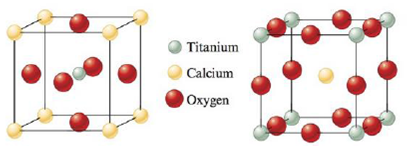Chapter 10, Problem 87E

Chapter
Section
Textbook Problem

Perovskite is a mineral containing calcium, titanium, and oxygen. Two different representations of the unit cell are shown below. Show that both these representations give the same formula and the same number of oxygen atoms around each titanium atom.Interpretation Introduction

Interpretation:

The given two Perovskite mineral representations are having same empirical formula has to be proved.

Concept Introduction:

• In packing of atoms/ions in a crystal structure, they are imagined as spheres. The two major types of close packing of the spheres in the crystal are – hexagonal close packing and cubic close packing.
• Cubic close packing has two types –Face-centered cubic close packing (FCC) and Body – centered cubic close packing (BCC).
• In edge-centered cubic unit cell, the eight corners is occupied by single atom and shared by 8 unit calls and the 12 edges are having 12 atoms and shared by 4 units center of the cube is occupied by one atom.
• In the In face-centered cubic unit cell, the eight corners is occupied by single atom and shared by 8 unit calls and the 6 faces are having 6 atoms and shared by 2 units, center of the cube is occupied by one atom.
Explanation

Explanation

Record the given data:

Perovskite mineral has Calcium, Titanium, and Oxygen atoms in below two type of unit cell arrangements shown in Fig 1.

Fig 1

The given unit cells diagram (Fig 1) is recorded as shown above.

To calculate the empirical formula of left side face-centered cubic Perovskite mineral.

Thenumberofatomsiscornersis,8corners×1/8Cacorner=1CaatomThenumberofatomsinfacesis,6faces×1/2Oface=3OatomsOne Ti in body center.

The empirical formula of left side face-centered cubic unite cell is CaTiO3

• The total atoms in faces are divided by number of faces in a cubic unit to give number of atoms in faces of one cubic unit and the number of atoms in corners are calculated by the total atoms in atoms in corners and divided total atoms in corners to give total atoms in corners in one cubic unite cell

Still sussing out bartleby?

Check out a sample textbook solution.

See a sample solution

The Solution to Your Study Problems

Bartleby provides explanations to thousands of textbook problems written by our experts, many with advanced degrees!

Get Started